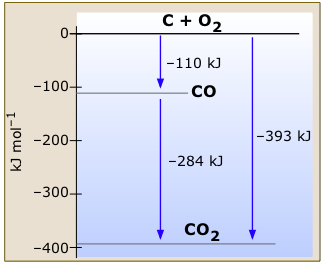# What is the importance of Hess's law to do thermodynamic calculations?

Jul 28, 2014

Hess's Law of Constant Heat Summation (or just Hess Law) states that regardless of the multiple stages or steps of a reaction, the total enthalpy change for the reaction is the sum of all changes.

Hess's Law is saying that if you convert reactants A into products B, the overall enthalpy change will be exactly the same whether you do it in one step or two steps or however many steps.

I will give you a simple example. You are on the ground floor of a five star hotel and wants to go to third floor. You can do so in three different ways (a) you can take the elevator directly from the ground floor to the third floor. (b) You can take the elevator from the ground floor to the second floor and then stop for a while at the second floor, take elevator from the second floor to third floor. (c) You can take the elevator from the ground floor to the first floor and then stop for a while at the first floor, take elevator from the first floor to third floor. It does not matter which way you may take , elevator is going to use the same amount of energy.
Let us take an example;
Carbon dioxide can be formed from carbon in two different ways.
When carbon combusts in an excess of oxygen, carbon dioxide is formed and 393.5 kJ of heat is released per mole of carbon.

C(s) + ${O}_{2}$(g) → ${C}_{1} {O}_{2}$(g) ΔH = -393.5 kJ

This overall reaction can also be produced as a two stage process:
Carbon combusts in limited oxygen producing carbon monoxide:
C(s) + ½${O}_{2}$(g) → CO(g) ΔH = -110.5 kJ

$$Carbon monoxide then combusts in additional oxygen:
CO(g) +1/2O2 (g)  → CO2(g)     ΔH = -283.0 kJ


These two equations can be added together to calculate ΔH for the overall reaction:

C(s) + ½${O}_{2}$(g) → CO(g) ΔH = -110.5 kJ
CO(g) + ½${O}_{2}$(g) → C${O}_{2}$(g) ΔH = -283.0 kJ
C(s) + ${O}_{2}$(g) → C${O}_{2}$(g) ΔH = -393.5 kJ